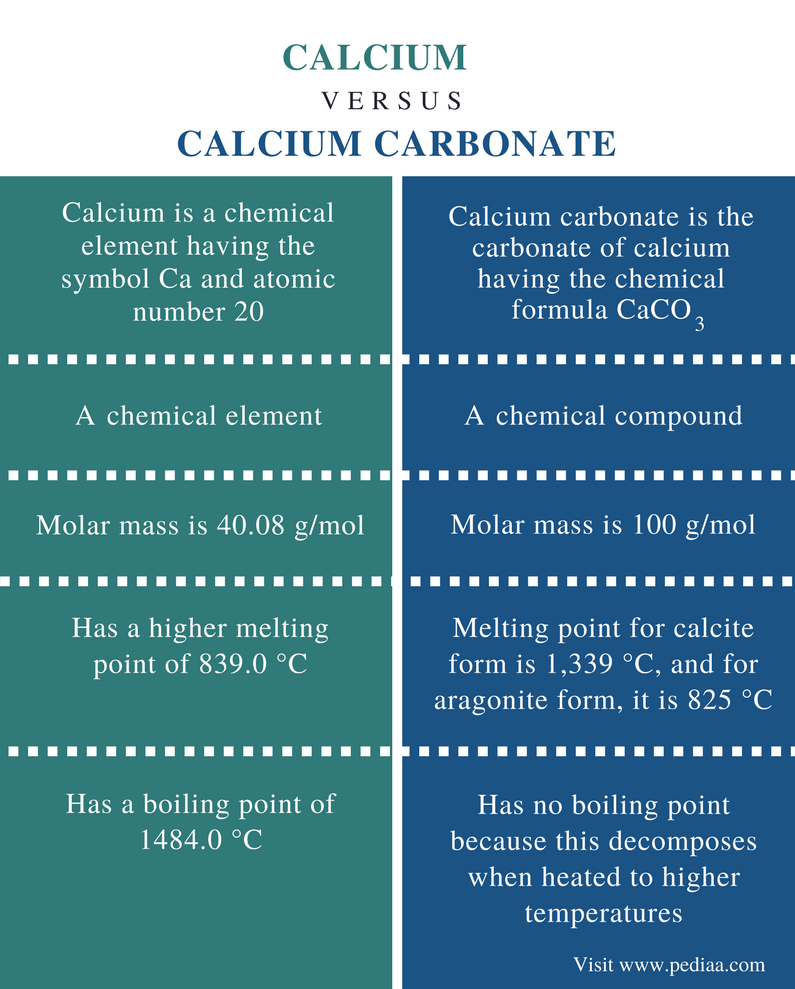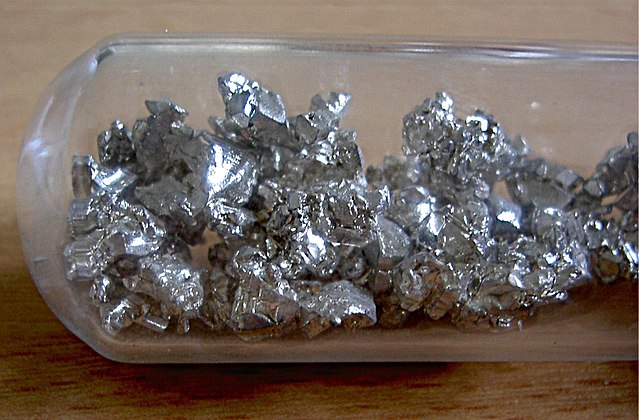# Difference Between Calcium and Calcium Carbonate

## Main Difference – Calcium vs Calcium Carbonate

Calcium is a chemical element having the symbol Ca. It is the 5th most abundant metal on earth’s crust. There are many naturally occurring compounds that contain calcium in their composition. Calcium has many applications as metal, calcium ion and calcium-bearing minerals. Calcium carbonate is the carbonate of calcium having the chemical formula CaCO3. It is an alkaline compound that forms calcium hydroxide when reacted with water. The main difference between calcium and calcium carbonate is that calcium is a chemical element whereas calcium carbonate is a chemical compound.

### Key Areas Covered

1. What is Calcium
– Definition, Chemical Properties, Occurrence, Uses
2. What is Calcium Carbonate
– Definition, Properties, Occurrence, Reactions
3. What is the Difference Between Calcium and Calcium Carbonate
– Comparison of Key Differences

Key Terms: Alkaline Metal, Calcium, Calcium Hydroxide, Calcium Ion, Calcium Carbonate, Chemical Element, Limestone, Radioactive Isotopes## What is Calcium

Calcium is a chemical element having the symbol Ca and the atomic number 20. Calcium is a group 2 element in the periodic table. It is an alkaline metal element since it forms alkaline (basic) compounds. Calcium metal has a silvery-white shine. It is a soft metal. Calcium easily tarnishes when exposed to air; it also reacts rapidly with water.

The molar mass of calcium is 40.08 g/mol. It is a metal having a higher melting point of 839.0 °C and a boiling point 1484.0 °C. At room temperature, it is in the solid state. Calcium atom has 20 electrons. The electron configuration of calcium is [Ar] 4s2. It has two valence electrons in the outermost s orbital. Therefore, calcium is an s block element, and the most stable ion it can form is the calcium divalent cation (Ca2+). However, calcium is less reactive than alkali metals (group 1 metals) and other alkaline earth metals.Figure 1: Calcium in an Argon Atmosphere

There are around 10 isotopes of calcium. It has both stable and radioactive isotopes. The most stable and abundant isotope among them is Ca-40. There are synthetic isotopes as well. Calcium is the 5th most abundant metal on earth.

Calcium occurs in sedimentary calcium carbonate minerals, limestone, dolomite, marble, chalk and many other mineral deposits. There are many uses of calcium as an element and calcium-containing compounds. Calcium metal is used to make metal alloys with aluminum. It is also used as a reducing agent in preparing other metals such as uranium. Limestone has many uses in controlling the acidity of water, soil, etc. In addition, calcium cations play an important role in physiology and biochemistry of organisms. Calcium is the most common mineral found in our body. It is required for the formation of bones and for the proper function of muscle contractions.

## What is Calcium Carbonate

Calcium carbonate is the carbonate of calcium, having the chemical formula CaCO3. This compound occurs in nature in different mineral deposits such as limestone, chalk, calcite, etc. It is the most common substance found in rocks in the form of calcite or aragonite (Limestone contains both these forms). Calcium carbonate can be found as white hexagonal crystals or powder. It is odorless and has a chalky taste.

The molar mass of calcium carbonate is 100 g/mol. The melting point of calcium carbonate for calcite form is 1,339 °C, and for aragonite form, it is 825 °C. It has no boiling point because calcium carbonate decomposes when heated to higher temperatures.Figure 2: Calcium Carbonate Rocks

Calcium carbonate is obtained through mining calcium-bearing mineral deposits. Pure calcium carbonate can be produced using a pure quarried source such as marble. Calcium carbonate can be produced by the reaction between calcium hydroxide (Ca(OH)2) and carbon dioxide (CO2).

Ca(OH)2   +   CO2    →   CaCO3     +     H2O

Calcium carbonate is an alkaline (basic) compound. It can react with acids releasing carbon dioxide gas. Calcium carbonate undergoes thermal decomposition, releasing carbon dioxide gas leaving calcium oxide. When calcium carbonate reacts with water, it forms calcium hydroxide.

## Difference Between Calcium and Calcium Carbonate

### Definition

Calcium: Calcium is a chemical element having the symbol Ca and atomic number 20.

Calcium Carbonate: Calcium carbonate is the carbonate of calcium having the chemical formula CaCO3.

### Nature

Calcium: Calcium is a chemical element.

Calcium Carbonate: Calcium carbonate is a chemical compound.

### Molar Mass

Calcium: The molar mass of calcium is 40.08 g/mol.

Calcium Carbonate: The molar mass of calcium carbonate is 100 g/mol.

### Melting Point

Calcium: Calcium has a higher melting point of 839.0 °C.

Calcium Carbonate: The melting point of calcium carbonate for calcite form is 1,339 °C, and for aragonite form, it is 825 °C.

### Boiling Point

Calcium: Calcium has a boiling point of 1484.0 °C.

Calcium Carbonate: Calcium carbonate has no boiling point because calcium carbonate decomposes when heated to higher temperatures.

### Conclusion

Calcium is a chemical element having the symbol Ca and the atomic number 20. Calcium forms different alkaline compounds; calcium carbonate is one such compound. Calcium Carbonate is a chemical compound having the chemical formula CaCO3. Therefore the main difference between calcium and calcium carbonate is that calcium is a chemical element whereas calcium carbonate is a chemical compound.

##### Reference:

1. “Water Treatment Solutions.” Lenntech Water treatment & purification, Available here.
2. “Calcium – Element information, properties and uses | Periodic Table.” Royal Society of Chemistry, Available here.
3. “CALCIUM CARBONATE.” National Center for Biotechnology Information. PubChem Compound Database, U.S. National Library of Medicine, Available here.

##### Image Courtesy:

1. “Calcium unter Argon Schutzgasatmosphäre” By Matthias Zepper – Self-photographed (Public Domain) via Commons Wikimedia
2. “Calcium carbonate rocks” By Ferdous – Own work (CC BY-SA 3.0) via Commons Wikimedia